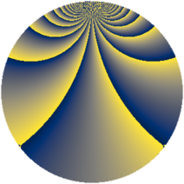# Properties

 Label 720.2.uLevel $720$ Weight $2$ Character orbit 720.u Rep. character $\chi_{720}(179,\cdot)$ Character field $\Q(\zeta_{4})$ Dimension $96$ Newform subspaces $1$ Sturm bound $288$ Trace bound $0$

# Related objects

## Defining parameters

 Level: $$N$$ $$=$$ $$720 = 2^{4} \cdot 3^{2} \cdot 5$$ Weight: $$k$$ $$=$$ $$2$$ Character orbit: $$[\chi]$$ $$=$$ 720.u (of order $$4$$ and degree $$2$$) Character conductor: $$\operatorname{cond}(\chi)$$ $$=$$ $$240$$ Character field: $$\Q(i)$$ Newform subspaces: $$1$$ Sturm bound: $$288$$ Trace bound: $$0$$

## Dimensions

The following table gives the dimensions of various subspaces of $$M_{2}(720, [\chi])$$.

Total New Old
Modular forms 304 96 208
Cusp forms 272 96 176
Eisenstein series 32 0 32

## Trace form

 $$96q + O(q^{10})$$ $$96q - 8q^{16} - 16q^{19} + 72q^{34} + 8q^{40} + 8q^{46} - 96q^{49} + 64q^{55} - 32q^{61} + 48q^{64} + 24q^{70} + 40q^{76} - 88q^{94} + O(q^{100})$$

## Decomposition of $$S_{2}^{\mathrm{new}}(720, [\chi])$$ into newform subspaces

Label Dim. $$A$$ Field CM Traces $q$-expansion
$$a_2$$ $$a_3$$ $$a_5$$ $$a_7$$
720.2.u.a $$96$$ $$5.749$$ None $$0$$ $$0$$ $$0$$ $$0$$

## Decomposition of $$S_{2}^{\mathrm{old}}(720, [\chi])$$ into lower level spaces

$$S_{2}^{\mathrm{old}}(720, [\chi]) \cong$$ $$S_{2}^{\mathrm{new}}(240, [\chi])$$$$^{\oplus 2}$$